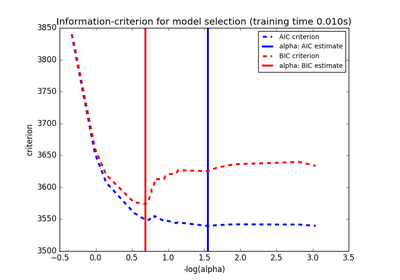# 3.2.4.2.1. `sklearn.linear_model`.LassoLarsIC¶

class `sklearn.linear_model.``LassoLarsIC`(criterion='aic', fit_intercept=True, verbose=False, normalize=True, precompute='auto', max_iter=500, eps=2.2204460492503131e-16, copy_X=True, positive=False)[source]

Lasso model fit with Lars using BIC or AIC for model selection

The optimization objective for Lasso is:

```(1 / (2 * n_samples)) * ||y - Xw||^2_2 + alpha * ||w||_1
```

AIC is the Akaike information criterion and BIC is the Bayes Information criterion. Such criteria are useful to select the value of the regularization parameter by making a trade-off between the goodness of fit and the complexity of the model. A good model should explain well the data while being simple.

Read more in the User Guide.

Parameters: criterion : ‘bic’ | ‘aic’ The type of criterion to use. fit_intercept : boolean whether to calculate the intercept for this model. If set to false, no intercept will be used in calculations (e.g. data is expected to be already centered). positive : boolean (default=False) Restrict coefficients to be >= 0. Be aware that you might want to remove fit_intercept which is set True by default. Under the positive restriction the model coefficients do not converge to the ordinary-least-squares solution for small values of alpha. Only coefficients up to the smallest alpha value (```alphas_[alphas_ > 0.].min()``` when fit_path=True) reached by the stepwise Lars-Lasso algorithm are typically in congruence with the solution of the coordinate descent Lasso estimator. As a consequence using LassoLarsIC only makes sense for problems where a sparse solution is expected and/or reached. verbose : boolean or integer, optional Sets the verbosity amount normalize : boolean, optional, default False If True, the regressors X will be normalized before regression. This parameter is ignored when fit_intercept is set to False. When the regressors are normalized, note that this makes the hyperparameters learnt more robust and almost independent of the number of samples. The same property is not valid for standardized data. However, if you wish to standardize, please use preprocessing.StandardScaler before calling fit on an estimator with normalize=False. copy_X : boolean, optional, default True If True, X will be copied; else, it may be overwritten. precompute : True | False | ‘auto’ | array-like Whether to use a precomputed Gram matrix to speed up calculations. If set to `'auto'` let us decide. The Gram matrix can also be passed as argument. max_iter : integer, optional Maximum number of iterations to perform. Can be used for early stopping. eps : float, optional The machine-precision regularization in the computation of the Cholesky diagonal factors. Increase this for very ill-conditioned systems. Unlike the `tol` parameter in some iterative optimization-based algorithms, this parameter does not control the tolerance of the optimization. coef_ : array, shape (n_features,) parameter vector (w in the formulation formula) intercept_ : float independent term in decision function. alpha_ : float the alpha parameter chosen by the information criterion n_iter_ : int number of iterations run by lars_path to find the grid of alphas. criterion_ : array, shape (n_alphas,) The value of the information criteria (‘aic’, ‘bic’) across all alphas. The alpha which has the smallest information criteria is chosen.

Notes

The estimation of the number of degrees of freedom is given by:

“On the degrees of freedom of the lasso” Hui Zou, Trevor Hastie, and Robert Tibshirani Ann. Statist. Volume 35, Number 5 (2007), 2173-2192.

Examples

```>>> from sklearn import linear_model
>>> clf = linear_model.LassoLarsIC(criterion='bic')
>>> clf.fit([[-1, 1], [0, 0], [1, 1]], [-1.1111, 0, -1.1111])
...
LassoLarsIC(copy_X=True, criterion='bic', eps=..., fit_intercept=True,
max_iter=500, normalize=True, positive=False, precompute='auto',
verbose=False)
>>> print(clf.coef_)
[ 0.  -1.11...]
```

Methods

 `decision_function`(\*args, \*\*kwargs) DEPRECATED: and will be removed in 0.19. `fit`(X, y[, copy_X]) Fit the model using X, y as training data. `get_params`([deep]) Get parameters for this estimator. `predict`(X) Predict using the linear model `score`(X, y[, sample_weight]) Returns the coefficient of determination R^2 of the prediction. `set_params`(\*\*params) Set the parameters of this estimator.
`__init__`(criterion='aic', fit_intercept=True, verbose=False, normalize=True, precompute='auto', max_iter=500, eps=2.2204460492503131e-16, copy_X=True, positive=False)[source]
`decision_function`(*args, **kwargs)[source]

DEPRECATED: and will be removed in 0.19.

Decision function of the linear model.

Parameters: X : {array-like, sparse matrix}, shape = (n_samples, n_features) Samples. C : array, shape = (n_samples,) Returns predicted values.
`fit`(X, y, copy_X=True)[source]

Fit the model using X, y as training data.

Parameters: X : array-like, shape (n_samples, n_features) training data. y : array-like, shape (n_samples,) target values. copy_X : boolean, optional, default True If `True`, X will be copied; else, it may be overwritten. self : object returns an instance of self.
`get_params`(deep=True)[source]

Get parameters for this estimator.

Parameters: deep : boolean, optional If True, will return the parameters for this estimator and contained subobjects that are estimators. params : mapping of string to any Parameter names mapped to their values.
`predict`(X)[source]

Predict using the linear model

Parameters: X : {array-like, sparse matrix}, shape = (n_samples, n_features) Samples. C : array, shape = (n_samples,) Returns predicted values.
`score`(X, y, sample_weight=None)[source]

Returns the coefficient of determination R^2 of the prediction.

The coefficient R^2 is defined as (1 - u/v), where u is the regression sum of squares ((y_true - y_pred) ** 2).sum() and v is the residual sum of squares ((y_true - y_true.mean()) ** 2).sum(). Best possible score is 1.0 and it can be negative (because the model can be arbitrarily worse). A constant model that always predicts the expected value of y, disregarding the input features, would get a R^2 score of 0.0.

Parameters: X : array-like, shape = (n_samples, n_features) Test samples. y : array-like, shape = (n_samples) or (n_samples, n_outputs) True values for X. sample_weight : array-like, shape = [n_samples], optional Sample weights. score : float R^2 of self.predict(X) wrt. y.
`set_params`(**params)[source]

Set the parameters of this estimator.

The method works on simple estimators as well as on nested objects (such as pipelines). The latter have parameters of the form `<component>__<parameter>` so that it’s possible to update each component of a nested object.

Returns: self :

## 3.2.4.2.1.1. Examples using `sklearn.linear_model.LassoLarsIC`¶Lasso model selection: Cross-Validation / AIC / BIC